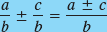### 11.7. Adding and Subtracting Fractions

From multiplying and dividing fractions, we now move on the operations of addition and subtraction.

#### 11.7.1. Similar Fractions

Similar fractions (also called like fractions) are those having the same (common) denominator.

NOTETo add or subtract similar fractions, combine the numerators and place them over their common denominator.

## Example 49:

These examples show the addition and subtraction of common and algebraic fractions having the same denominators.#### 11.7.2. Least Common Denominator

The least common denominator, or LCD (also called the lowest common denominator), is the smallest expression that is exactly divisible by each of the denominators. Thus the LCD must contain all the prime factors of each of the denominators. The common denominator of two or more fractions is simply the product of the denominators of those fractions. To find the least common denominator, drop any prime factor from one denominator that also appears in another denominator.

## Example 50:

Find the LCD for the two fractionsand.

Solution: Factoring each denominator, ...

Get Technical Mathematics, Sixth Edition now with the O’Reilly learning platform.

O’Reilly members experience books, live events, courses curated by job role, and more from O’Reilly and nearly 200 top publishers.